Homework Help Question & Answers

For the relation R(O,P,X,Y,E, S) FDs={ O->P, XY->O, OE->S, EY->X, PY->X}. 1.Classi...

for the relation R(O,P,X,Y,E, S) FDs={ O->P, XY->O, OE->S, EY->X, PY->X}. 1.Classify each functional dependency 2. Decompose the relation into BCNF relation. 3. Determine whether each of the decompositions has the lossless join property with respect to F and conclude for the whole decomposition. 4. Determine whether each of the decompositions has the dependency preserving property with respect to FD and conclude for the whole decomposition

Dear student, according to the question, the solution is as follows: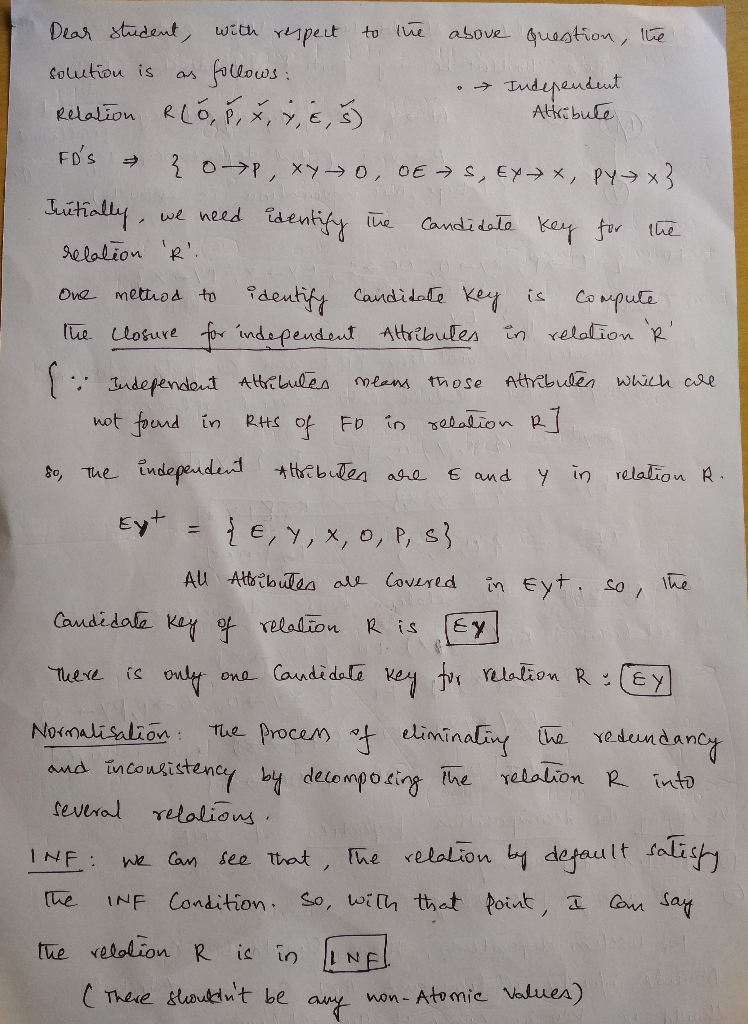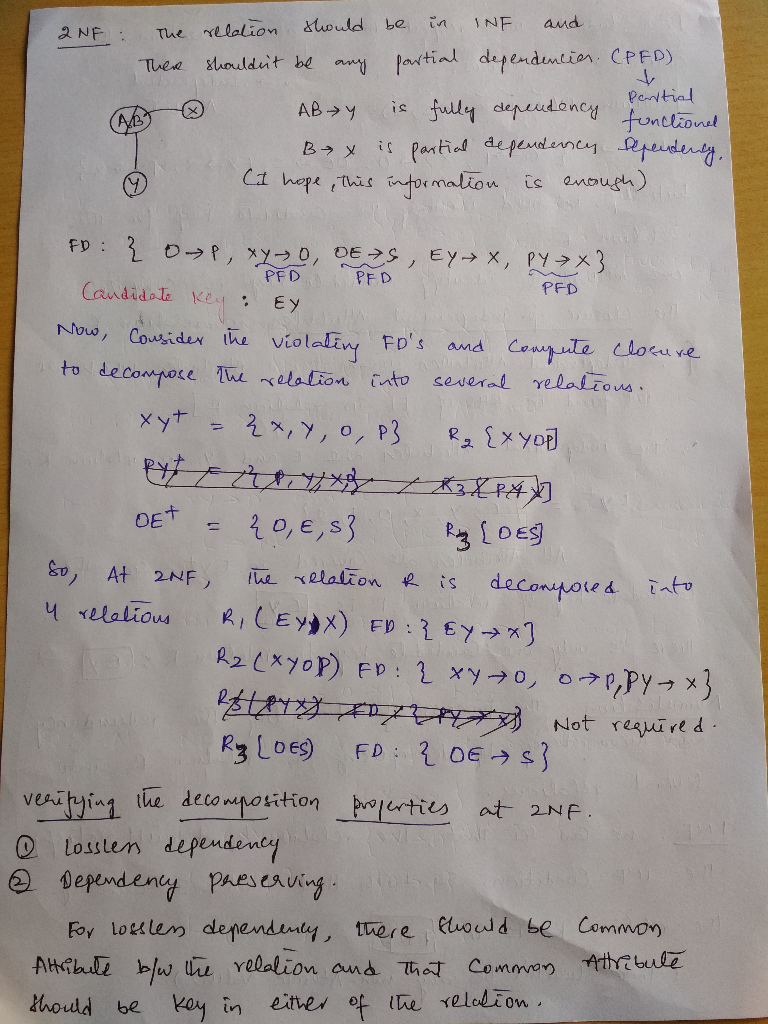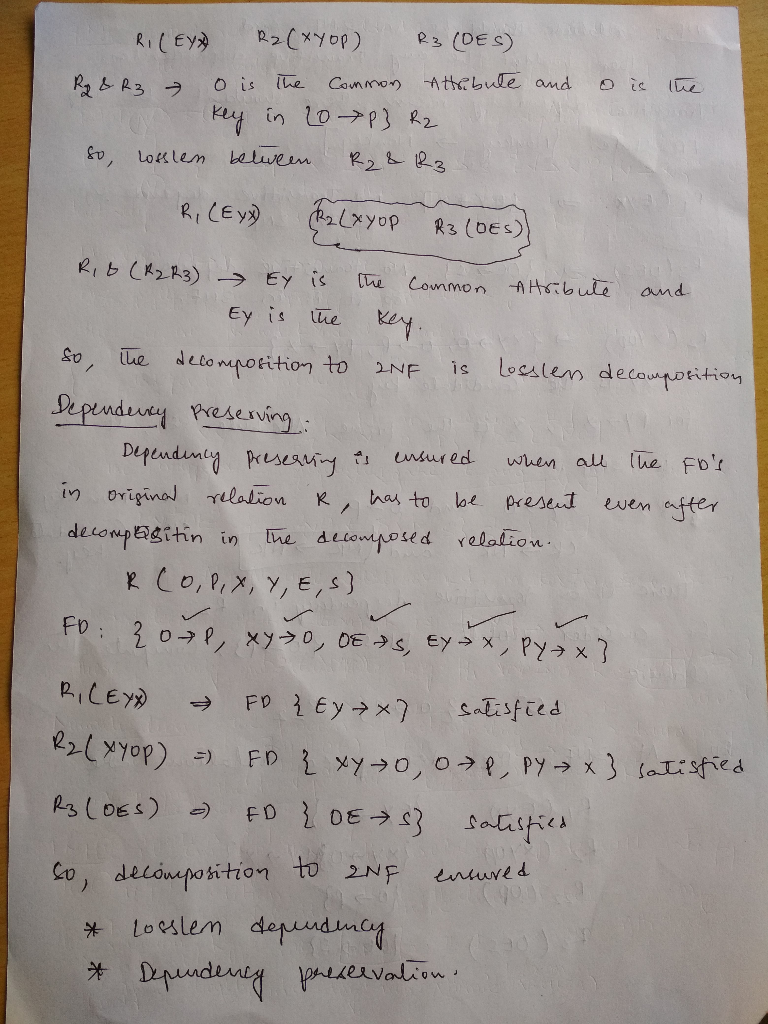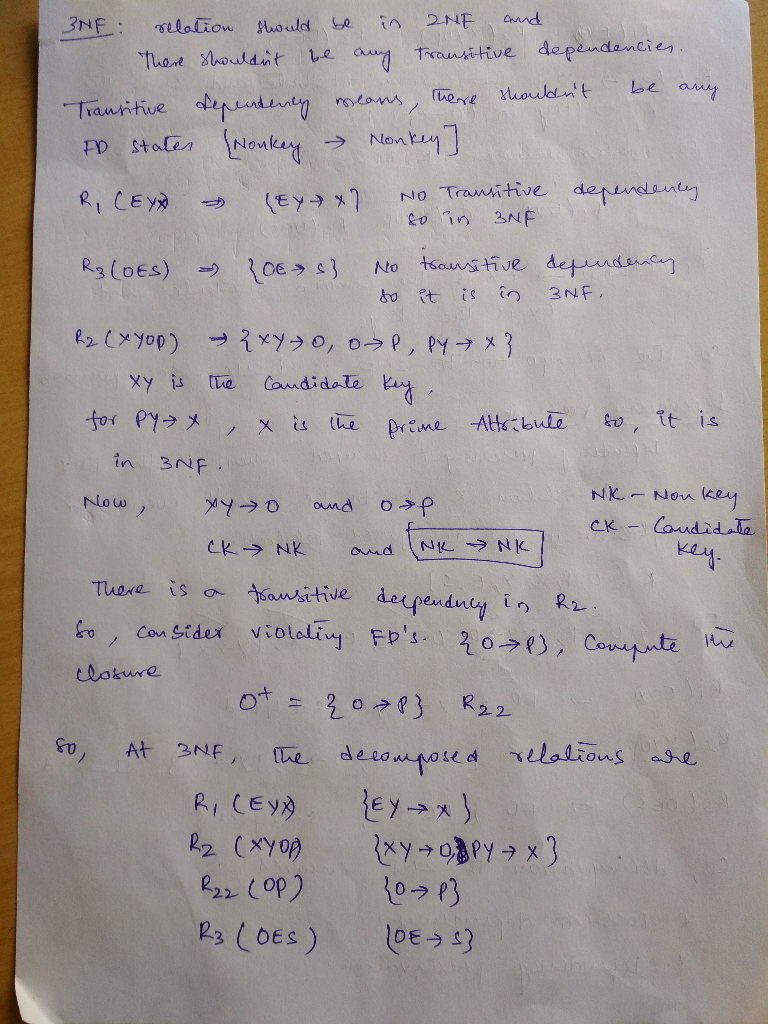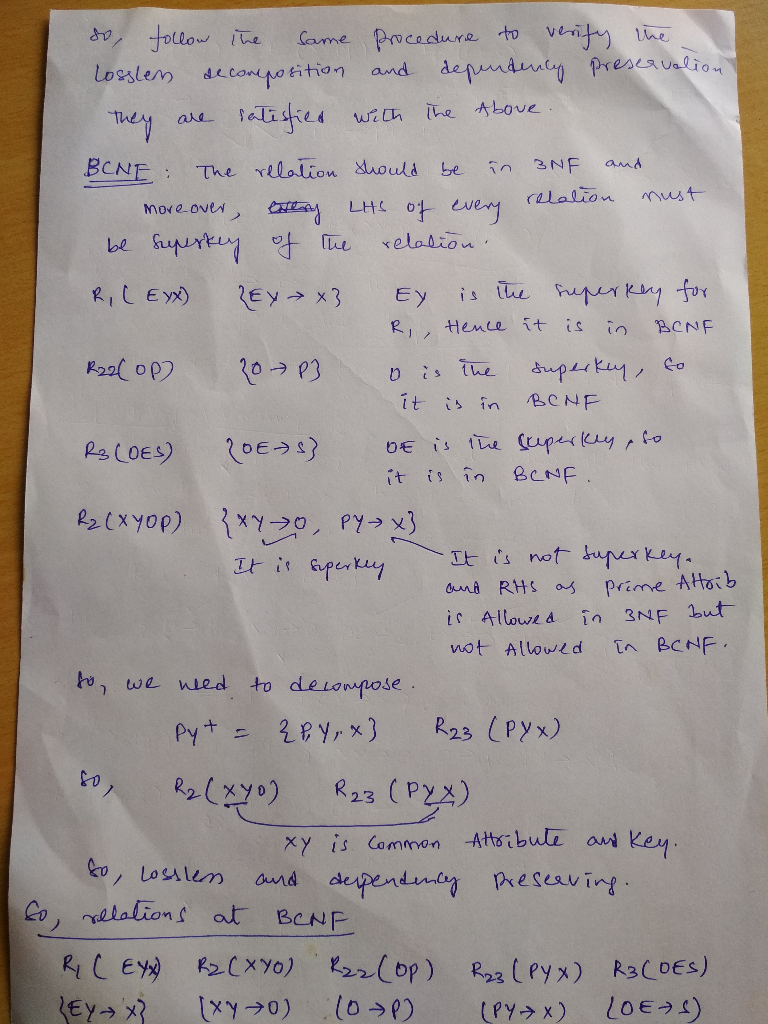So, finally, the relation R (O,P,X,Y,E,S) has been decomposed into 5 relations to meet the requirements of BCNF.

R1(EYX), R2(XYO), R3(OP), R4(PYX), R5(OES)

Thank you, All the best.

If at all any doubts, feel free to contact through the comment section

Add Answer of: For the relation R(O,P,X,Y,E, S) FDs={ O->P, XY->O, OE->S, EY->X, PY->X}. 1.Classi...
More Homework Help Questions Additional questions in this topic.

• In each of the following, a relation and a set are given. Write yes if the relation is an...

Need Online Homework Help?

Get FREE EXPERT Answers
WITHIN MINUTES
Related Questions